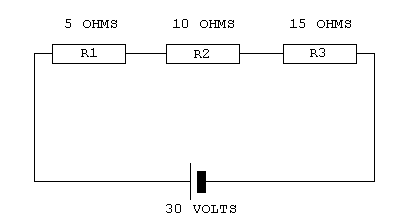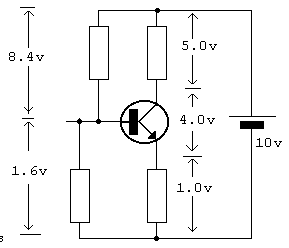Home > Electronics Tutorials > Direct Current (DC) Theory > Voltages in a Circuit Tutorial

Direct Current (DC) Theory - Electronics Teaching

# Voltages in a Circuit TutorialEnsure that you understand Ohms Law before tackling this,

In the diagram the total series resistance = 30 ohms.

The current flowing is 30 volts/30 ohms = 1 amp.

Thus the current through the 5 ohm resistor is 1 amp.

Therefore the voltage across the 5 ohm is 1 amp x 5 ohm = 5 volts.

Similarly the voltage across R2 is 10 volts.

The voltage across R3 is 15 volts.

If you add these three voltages 5 + 10 + 15 = 30 volts.

This is the same as the battery voltage.

Add up both sets of voltage in the transistor circuit diagram.

They both add up to 10 volts , which is the battery voltage.Note: To report broken links or to submit your projects, tutorials please email to Webmaster

 Discover